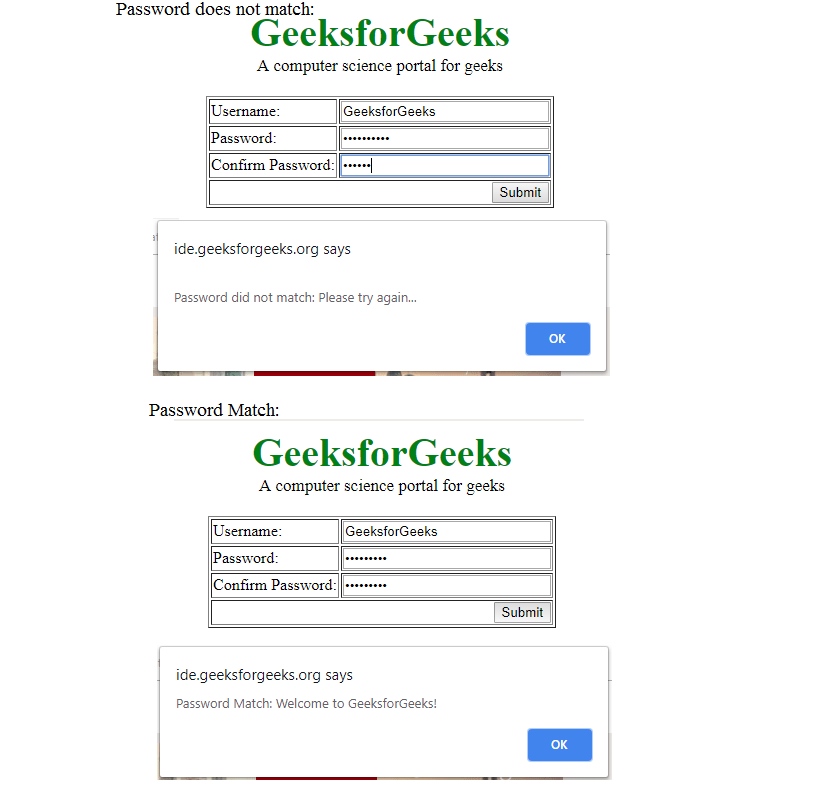GeeksforGeeks App
Open AppBrowser
Continue

 ```<``html``>``    ``<``head``>``        ``<``script``>``         ` `            ``// Function to check Whether both passwords``            ``// is same or not.``            ``function checkPassword(form) {``                ``password1 = form.password1.value;``                ``password2 = form.password2.value;`` ` `                ``// If password not entered``                ``if (password1 == '')``                    ``alert ("Please enter Password");``                     ` `                ``// If confirm password not entered``                ``else if (password2 == '')``                    ``alert ("Please enter confirm password");``                     ` `                ``// If Not same return False.    ``                ``else if (password1 != password2) {``                    ``alert ("\nPassword did not match: Please try again...")``                    ``return false;``                ``}`` ` `                ``// If same return True.``                ``else{``                    ``alert("Password Match: Welcome to GeeksforGeeks!")``                    ``return true;``                ``}``            ``}``        ````        ``<``style``>``            ``.gfg {``                ``font-size:40px;``                ``color:green;``                ``font-weight:bold;``                ``text-align:center;``            ``}``            ``.geeks {``                ``font-size:17px;``                ``text-align:center;``                ``margin-bottom:20px;``            ``}``        ````    ````    ``<``body``>``        ``<``div` `class` `= ``"gfg"``>GeeksforGeeks``        ``<``div` `class` `= ``"geeks"``>A computer science portal for geeks``        ``<``form` `onSubmit` `= ``"return checkPassword(this)"``>``        ``<``table` `border` `= ``1` `align` `= ``"center"``>``            ``<``tr``>``                ````                ``<``td``>Username:``                ``<``td``><``input` `type` `= ``text` `name` `= name ``size` `= ``25````            ````            ``<``tr``>``                ````                ``<``td``>Password:``                ``<``td``><``input` `type` `= ``password` `name` `= ``password1` `size` `= ``25````            ````            ``<``tr``>``                ````                ``<``td``>Confirm Password:``                ``<``td``><``input` `type` `= ``password` `name` `= ``password2` `size` `= ``25``>``            ````            ``<``tr``>``                ``<``td` `colspan` `= ``2` `align` `= ``right``>``                ``<``input` `type` `= ``submit` `value` `= ``"Submit"``>``            ````        ````        ````    ````                    `